# What is an LC low pass filter

## Passive electrical filters

Passive band-pass and band-stop filters, the circuits of which have RC and RL elements, are shown under this title. A filter used in entertainment electronics is the sound adjustment network that is used in both passive and active circuit variants. Better filter properties are achieved with T and Pi filter circuits, which are shown in their basic circuits. A large filter group is made up of LC basic half-links, which can then be found as single-link LC basic chains in many LC band filters for selective amplifiers. The Boucherot circuit as a resonance transformer and the Collins filter for power adjustment are also described here as special LC filters.

Electronic filter circuits influence the frequency range of supplied electrical input signals. An electrical filter has at least one characteristic pass band and stop band. A cut-off frequency is defined for the range transition at which the amplitude of the output signal is attenuated by a = 3 dB. The logarithmic measure means that the output amplitude is smaller than the input amplitude by a factor of 1 / √2 = 0.7071 and corresponds to a gain of V = −3 dB. The filter characteristic can be described as attenuation or transmission behavior, which are mutually reciprocal. The transfer behavior is almost always given as the ratio of the output to the input variable. In the case of passive filter circuits, the output amplitude is always less than or equal to the input amplitude.

Simple filters, which include RC and RL low and high passes, only have one cutoff frequency fg.
The following applies to fg: Ua / Ue = 1 / √2 = 0.7071.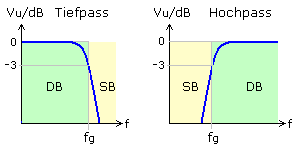### Low pass filter

All frequencies up to the cutoff frequency, sometimes referred to as the cutoff frequency, are passed through to the output with almost no attenuation. The signal amplitudes of the higher frequencies are increasingly attenuated.

### High pass filter

The frequencies above the cut-off frequency pass through the filter and are available unattenuated at the filter output. The further you get from the corner frequency into the range of lower frequencies, the more the amplitudes are weakened. DC voltage components are completely blocked.

### Band pass filter

Combined filter circuits with resonance circuits or independent RC and RL groups form bandpass filters or bandstop filters. These filters have their characteristic extreme value at the center frequency fo. In addition to the center frequency, there is a lower fgu and upper fgo limit frequency at the 3 dB points. The center frequency is calculated as a geometric mean value from the limit frequencies. Another characteristic filter variable is the bandwidth B, the difference value between the upper and lower limit frequency. The basic filter curves with the pass-through DB and cut-off range SB and the position of the cut-off frequencies are shown.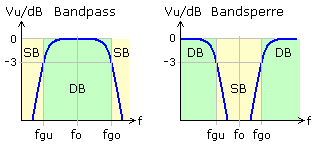A measuring band filter AES17-1998 standardized by the Audio Engineering Society is used in digital audio technology. It is a bandpass filter with an amplitude constancy of ± 0.1 dB in the range from 10 Hz to 20 kHz and an attenuation of at least 60 dB at 24 kHz.

If the amplitude-frequency response of the band-pass or band-stop filters is shown with a linearly divided amplitude axis, then the transmission curve is similar to the profile of a bell curve. The filters are therefore also called bell filters (English peak filters), which also include series and parallel resonant circuits.

### Octave filter and third octave filter

These are bandpass filters whose cut-off frequencies are in a fixed ratio. The transfer functions are mostly standardized and the filters have very steep slopes. In audio technology, the octave corresponds to a doubling of the frequency. The bandwidth of three consecutive third-octave filters is one octave. By defining a cut-off frequency, the associated second cut-off frequency is calculated as follows.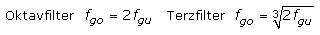### Band reject filter (notch filter, band reject filter)

The bandstop has a behavior that is mirrored to the bandpass. The filter selects a certain, often very narrow frequency band. In principle, these are high and low passes connected in parallel. All frequencies up to the lower limit frequency and from the upper limit frequency pass without attenuation. The frequency range in between is increasingly weakened towards the center frequency. A bandstop filter with a very narrow frequency band, steep edges and high attenuation is called a notch filter. If it is a series resonant circuit, one speaks of a trap (trap filter) or suction circuit.

### All-pass filter

The only exception with this type of filter is the signal amplitude in the entire frequency range. The filter has a cutoff frequency. In the working range below the limit frequency, the signal group delay is independent of the frequency. The output signal experiences a frequency-dependent phase shift. The all-pass filter works as a phase shifter or delay element and thus passes the input signal on to the output unchanged but with a time delay. A comb filter is the interconnection of several coordinated all-pass filters.

### Slope

The edge steepness is a parameter and a measure of the change in the transmission amplitude in the blocking range. Since the transition from the pass band to the stop band does not take place with a sharp bend and is linear, it is necessary to specify a reference frequency. The characteristic curve shown in logarithmic graduation is linear enough from the cut-off frequency. The slope or attenuation is then given as a parameter per octave, the frequency doubling or decade, the frequency tenfold. Filters of the nth order have an attenuation of n · 6 dB / octave and n · 20 dB / decade with n as an integer greater than zero. The higher the ordinal number, the more likely it is that a disturbing ripple can occur in the pass band.

### Selectivity - goodness

In addition to the useful frequencies, small portions of undesired frequency ranges also reach the filter output. The selectivity is the quotient of the output amplitude or the transfer factor in the pass band at the center frequency in relation to the transfer factor from a specified interference frequency. Filters with a low bandwidth are characterized by a high filter quality. The nameless quality factor Q is calculated as Q = fo / B = fo / (fgo - fgu).

### Operational damping

With passive filters, the output signal is always smaller than the input signal. The operational attenuation is the ratio of the input power to the output power in relation to the passband. In active filter circuits, this can be compensated for by a linear amplification.

### Phase delay

If a signal with a certain frequency passes through a filter or an electronic assembly, there may be a time delay, which can then be measured as a phase shift between the input and output signal. The signal takes a certain amount of time because it moves from input to output at a finite speed. In a stationary system, the phase delay is the time it takes for the output signal to reach the phase state of the input signal.The phase delay can be represented as a function of the frequency and is then calculated using the radian measure of the phase angle. With monofrequency signals there is only a phase shift and no distortion. If the phase delay of a transmission system is constant in the frequency range under consideration, the signal is transmitted without distortion. A high or low pass does not meet this phase condition over its entire frequency range, so that signals with many harmonics are not transmitted without distortion.For an RC low-pass filter, the frequency-dependent phase delay was entered in the phase-frequency diagram. Below the cut-off frequency, the phase delay is almost constant and a mixed signal made up of three sinusoidal frequencies experiences only one phase shift. With the start of the blocking range, the phase delay is no longer constant and the mixed signal with ten times the frequency values ​​shows a strongly distorted output signal.The dependence of the phase delay on the frequency is called phase distortion. Their influence is negligible in audio technology, as the human ear is very insensitive to it. In television technology, phase distortions make themselves felt as changes in color tone, to which human eyes are very sensitive.

### Group delay

If a narrow-band signal group, for example a modulation signal, passes through a filter or an electronic assembly, the signal arrives at the output with a time delay. The group delay is the time with which the envelope of the overall signal appears delayed at the output. The group delay is calculated from the derivation of the phase response at the respective frequency and indicates the slope of the phase response. In a frequency range with a linear phase response, the group delays are constant. A narrow-band signal that does not exceed this frequency range of the system (band filter) is transmitted without distortion.The picture shows a simple resonant circuit filter for an amplitude-modulated intermediate frequency signal of analog broadcast technology. The IF frequency used is 455 kHz and the bandwidth of the AM signal is set to 9 kHz. The resonant circuit has a bandwidth of 11 kHz and shows a largely linear phase response between the limit frequencies in the Bode diagram. In this area, the gradient of the straight lines drawn in red, and thus also the group delay, are constant. The AM IF signal appears delayed and undistorted at the filter output.

The group speed on lines is between 10,000 km / s and almost the speed of light. A delay of a few seconds is therefore noticeable for radio links via satellite. In the terrestrial telecommunications network, transit times of up to 250 ms are permitted in each transmission direction. The disruptions in the flow of calls that occur are less than 10%. The frequency-dependent group delay leads to group delay distortions. They are related to a fixed reference frequency or a frequency with a smaller group delay. The distortions could falsify individual speech sounds in the audio range, since higher speech frequencies arrive at the receiver before lower ones. In the audio sector, these interferences are almost always negligible, but in image transmission they have to be compensated for by special measures.

### Passive band pass and band stop filters

The simplest basic circuits include the RC and RL voltage dividers. These high and low passes have a component that is dependent on the frequency and are therefore first order passes or filters. The series connection of HP and TP results in a band pass if the cut-off frequency of the TP is equal to or greater than that of the HP. A modified parallel connection of HP and TP leads to the bandstop filter. Both filters do not have such good filter properties because, like first-order filter passes, they only achieve a slope of 20 dB per decade or 6 dB per octave. With two independent storage elements, it should actually be a 2nd order filter. Disadvantages arise from the mutual stress when connecting the individual passes directly to the filter. An excessively high internal resistance of the input signal source and an output load also have a negative effect on the maximum level and shift the limit frequencies.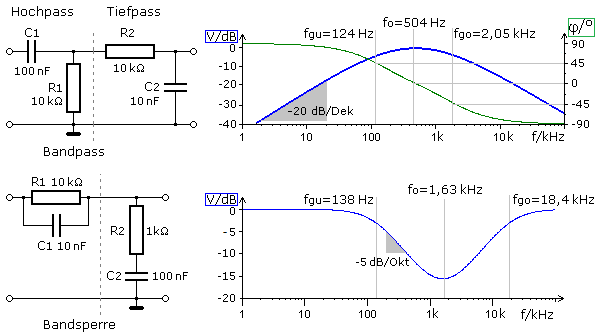In the band pass the cutoff frequency for the high pass is 159 Hz and for the low pass 1.592 kHz. The center frequency fo = 504 Hz can be read from the Bode diagram of the circuit simulation. It is also calculated as a geometric mean from the unloaded limit frequencies of the HP and TP. The mutual loading results in other limit frequency values ​​for the bandpass whose geometric mean again results in the same center frequency.

With the bandstop filter, the cut-off frequency of the TP should be lower than that of the HP. A real parallel connection of both passes as in the following circuit would be more optimal. The two output voltages are combined at high resistance with the help of an adder to form the common filter output. The slope is greater and does not reach the 12 dB / octave of a 2nd order filter here either. The cut-off frequency of the HP is 15.92 kHz and that of the TP 159 Hz and deviates less strongly in this band-stop filter due to the better decoupling. Optimal decoupling of the individual passes can be achieved using non-inverting operational amplifiers. Elsewhere, the loaded RC bandpass is described with detailed mathematical information.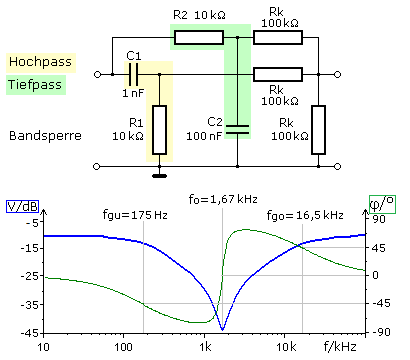### Sound Adjustment Network - Shelving Filter

Treble and bass adjusters are used in the audio sector of home electronics to influence the sound. In the simplest case, they are passive RC circuits, where the amplitude frequency responses are influenced with potentiometers. If the filters in the feedback branch are between two amplifier stages, the adjustable frequency range can also be dampened or amplified in a controlled manner. The following circuit shows a passive universal fan network with a height and depth adjuster, also called a cow or dovetail equalizer. It is an industrially used circuit extract that is similar to the classic fan-shaped equalizer. Here, the frequency-dependent bridge branches act as adjustable high and low passes. The diagram shows the amplitude-frequency response for different settings of the treble and bass potentiometers. With the low pass (TP) the useful signal is tapped parallel to the capacitor, with the high pass (HP) parallel to the effective resistance. The left branch of the bridge acts as TP, the right branch of the bridge as HP.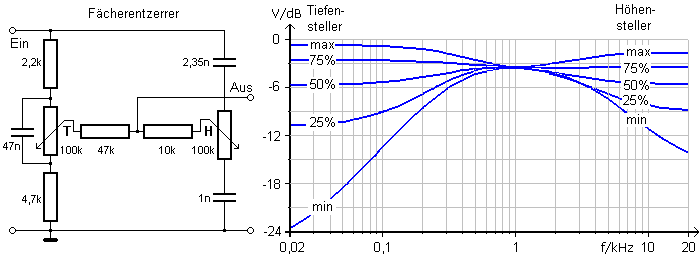If the slider of the depth adjuster T is at the top (maximum), the upper resistor with the capacitor in the left branch can be viewed as a low pass. The capacitor has a high resistance value for low signal frequencies. At 100 Hz it is around 33 kΩ, at 1 kHz it is 3.4 kΩ. In the parallel connection, the capacitor determines the effective partial resistance and, depending on the slider position, more or less signal amplitude is coupled out via the bridge branch to the output. In the lower position (minimal) the depth adjuster decouples the signal amplitude at the lower resistor. With this resistor, the capacitor forms an RC-HP. The high frequency components of the input signal are hardly attenuated by the left capacitor and are available as a relatively large signal amplitude at the lower resistor. The left branch of the bridge, adjustable with the potentiometer, dampens the low frequency ranges of the input signal.

If the wiper of height adjuster H is at the top (maximum) in the right part of the bridge, it forms a high pass with the upper capacitor. The low-frequency signal components present in the input signal are strongly attenuated by the upper capacitor, while the higher frequencies at the potentiometer's rail resistance lead to a high signal amplitude. At a frequency of 1 kHz, the upper capacitor has a resistance value of around 68 kΩ and at 5 kHz it is around 13.5 kΩ. As the signal frequency increases, the capacitor attenuates less and less and the signal amplitude that can be removed by the grinder increases with the position unchanged. Depending on the position of the slider, a more or less large signal amplitude is coupled out to the output. The right branch of the bridge only slightly attenuates the higher signal components present in the input signal. In the lower wiper position (minimal) the signal is decoupled at the lower capacitor and the height adjuster forms a low pass with this capacitor. In this position, the high frequency ranges are most attenuated in the decoupled signal.

Neither of the two sides of the bridge are pure high or low passes. Depending on the position of the grinder, both properties are more or less effective. A simple limit frequency calculation is not possible here.

### T and Pi filter circuit

When connecting two identical passports, there are two circuit variants that look like the letters T or Pi. Both circuit variants are equivalent for RC and RL passes. In the lower and middle frequency range, RC filters are preferred wherever possible, since coils are more expensive, larger and, compared to capacitors, have a lower quality. T and Pi filter circuits in LC technology form filters of a higher order with steeper edges and a better separation between the pass band and stop band. The picture shows some simple basic types.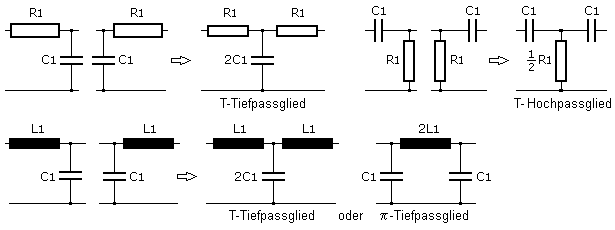The following circuit shows the application of a pi filter. It is a presence filter to improve speech intelligibility. The circuit was used in a Hi-Fi receiver from SABA. According to the telephony bandwidth, the voice band is between 300 Hz and 3.4 kHz. This filter works between 1.2 kHz ... 5.5 kHz with the maximum passage at a center frequency of 2.6 kHz. In the receiver, the filter is located in the feedback branch of two transistors, which reverses the attenuation into an amplification. Viewed from the output, the 22 nF capacitor in the bridge connection with the 5.6 kΩ acts as a high pass on the input. Seen from the input, this capacitor with the 1.8 kΩ represents a high-pass filter. With it, the low-pass filter in the output branch loses its influence at higher frequencies.Another example shows the use of a double-T or notch filter made up of RC high-pass and low-pass elements. It is a 16 kHz block to block the charge pulse in telephone traffic, which could disrupt the modem operation on the PC. The center frequency determined by simulation is 15.8 kHz. With the same resistance and capacitor values ​​in the through branch, the resistance connected to ground has half the value and the capacitor double the value.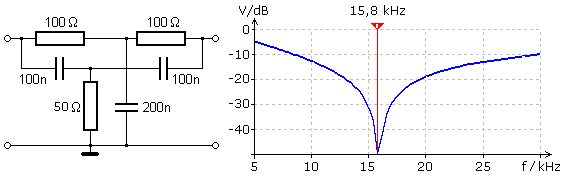### Remote feeder (bias tea)

The remote feeder, a T-shaped filter in HF technology, separates a DC supply or switching voltage from HF signals. There are crossovers that are in English. Linguistic area are referred to as bias tee and are often used in antenna technology in front of RF amplifiers and preamplifiers. In the circuit above, the DC supply voltage cannot reach the HF output via the capacitor C1. The high inductive resistance blocks the HF downwards, but is ineffective for DC and low frequencies. The DC voltage is coupled in or out between C2 and L and is separated from ground by C2, while the remaining HF components are short-circuited to ground.With suitable dimensioning of the components, this crossover can also separate a mixed RF input signal in the same way. The second circuit shows the functionality created with the help of a simulation. The distance between the HF1 frequency of 10 MHz and the HF2 frequency of 100 kHz is far enough away for easy filtering. The 75 Ω commonly used in antenna technology are used as input and output resistances.

### Higher order filters - passive LC filters

The filters presented so far do not have particularly steep slopes. With only one frequency-dependent component, the basic elements have a maximum slope of 6 dB per octave or 20 dB per decade. LC filters with two components determined by the frequency show a better filter effect with 12 dB per octave or 40 dB per decade. Without additional ohmic resistors, the basic attenuation in the pass band is much lower. In addition to these advantages, the resonance behavior of the simple LC elements can be problematic. As shown for the loudspeaker crossovers, the filters must be adapted to the connected load resistance.

A coil and a capacitor connected in series form a series resonant circuit with a resonance frequency and two cutoff frequencies. The amplitude curve of the output signal, which is tapped in parallel to the capacitor, decreases after the upper limit frequency. This circuit variant is comparable to a low-pass filter. Between the cut-off frequencies lies the resonance frequency, which can be calculated using the Thomson formula and which becomes the filter frequency. There is an excessive voltage increase at the reactance, which depends on the circuit quality. If one assumes ideal behavior for L and C, the circular quality is determined by the internal resistance of the source and the resistance of the load. With an adapted load, the increase is avoided and the slope remains for the 2nd order filter.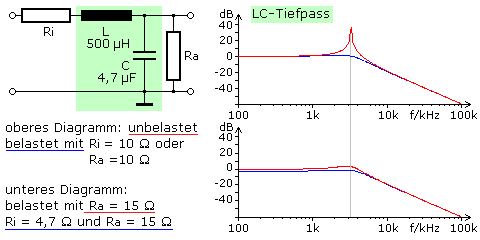If the positions of L and C are swapped in the circuit and the output signal is tapped parallel to the coil, then the frequency range is strongly attenuated up to the cutoff frequency and the circuit acts as a high-pass filter. The amplitude diagrams are mirror-symmetrical to the center (resonance) frequency for the same load cases.

An LC parallel resonant circuit is characterized by excessive voltage and strong damping outside the bandwidth range at resonance frequency. The bandwidth is determined by the load caused by the source impedance and the load impedance of the subsequent stage. The quality of the coil is determined by the ohmic wire resistance and is usually much higher. As the load increases, the bandwidth B increases and the filter quality Q decreases. It is calculated with Q = fo / B, from the ratio of the center frequency to the bandwidth. With decreasing quality, the slope also becomes significantly smaller.The resonant circuit connected in series to the signal path results in a bandstop filter. The circular quality is determined in the simulation by the inserted wire resistance of the coil to Q = 140. A smaller output resistance places a greater load on the filter, so that the quality decreases and the bandwidth increases. At the same time, the edge steepness in the transition area from the passage to the blocked area decreases.

An improved method is created through the use of T and Pi filter elements. The basic components of the LC filter circuits are called half-links and are made up of a series and a transverse two-pole. The product of the series and transverse impedance should be independent of frequency. The following picture shows the four most important basic half-links. A coil in the series branch, like the capacitor in the shunt branch, leads to the low pass. For a high pass, the capacitor is in the series arm and the coil is in the shunt arm. Likewise, a series resonant circuit is complemented lengthways with a parallel resonant circuit across the bandpass. If the oscillating circuits are exchanged for one another, a bandstop is created.

### Duality invariant

Two circuits are dual to each other if the (complex) alternating current resistance of one is proportional to the (complex) conductance of the other. The constant of proportionality is called the duality invariant and has the dimension Ω².### Basic half-members of passive LC filter circuitsWhile a high and low pass is relatively easy to calculate mathematically, the calculation of a band pass and bandstop is demanding. The main equations are from the book of D. Stoll: Introduction to communications technology, AEG-Telefunken 1979 taken. The following formulas are given for the basic elements high and low pass: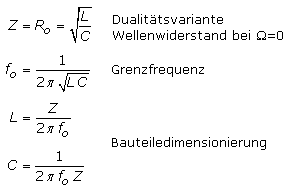The determining equations apply to the basic half-member LC bandpass: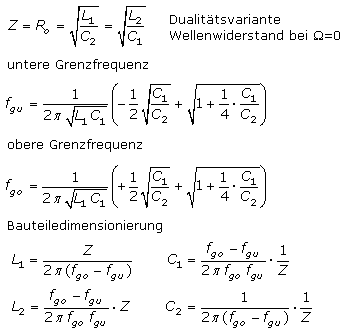The determining equations apply to the basic half-element LC bandstop filter: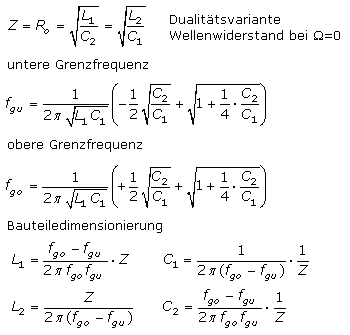### Resonance transformer - Boucherot circuit

An LC filter half-element operated as a series resonant circuit in resonance with a load resistor connected in parallel to the capacitor is referred to as a Boucherot circuit. A constant current flows through the load resistor when there is resonance, and since the circuit can transmit voltage, current and power like a transformer, it is also known as a resonance transformer. Among other things, it is used to adjust the power between the internal resistance of a source and the connected load.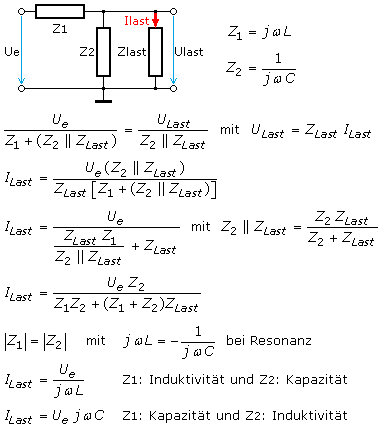A simulation circuit was investigated with Z1 as inductance L = 800 mH, and the capacitance Z2 at C = 12.5 μF at the resonance frequency of 50 Hz. With a constant sinusoidal effective input voltage of 10 V, the input currents Ie, Load currents Ia and voltages Ua determined at the load. The table shows the mathematically predicted constant load current.

Circuits with the resonance transformer can be found in the control of energy-saving lamps and electronically controlled fluorescent tubes as well as the operation of CCFL cold cathode tubes for the background lighting of TFT-LCD flat screens. As long as a fluorescent tube has not ignited, its load resistance is very high and, as can be seen from the table values, the resonance transformer itself provides the necessary high ignition voltage.

In high-frequency technology and in transmitting and receiving devices, resonance transformers can be used to adjust the power between different function blocks. The impedance of the source, simplified to its internal resistance R1 in the following example, is parallel to the LC series circuit. The load impedance, simplified to an ohmic resistor R2, is parallel to the capacitor. With power adjustment, the left side with its internal resistance gives off as much power to the load R2 as an imaginary source on the right with the load internal resistance R2 would give to the left side, where then R1 can be seen as the load.In the following example, in a VHF IF filter chain, the output of a low-impedance IF source with R1 = 50 Ω is adapted to a high-impedance subsequent stage with R1 = 2.2 kΩ with the greatest possible power. The resonance frequency is 10.7 MHz. With the derived formulas, the inductance is calculated as 4.9 μH and the capacitance as 44 pF. The performance values ​​were determined using a circuit simulation.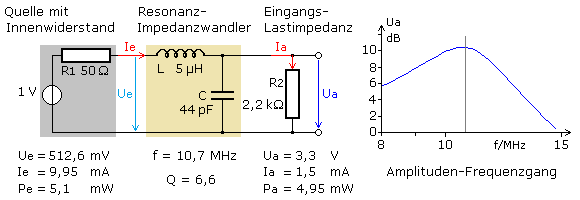### Collins filter

The less the values ​​of the source and load impedance differ, the lower the operational quality and the resonance transformer has a large bandwidth. Because the LC filter can transform the impedances in both directions, two differently dimensioned LC low-pass filters can be interconnected to form a PI filter. The arrangement is called a PI coupler or, after the Rockwell Collins company, a Collins filter, which it used in their radios. With the filter, impedance adjustments between source and load are also possible for higher quality values. Tunable Collins filters are often used in RF technology for low-reflection, power-adjusted signal transmission between the transmitter output stage and antenna. A maximum power transmission should take place at the operating frequency and the attenuation outside the bandwidth should be as high as possible.

Two resonance transformers connected in series with the inductors form a Collins Pi filter. In relation to the signal, the input and output loads are parallel to the capacitors. In this way, the load resistance on one side is transformed down to an imaginary small auxiliary resistance and, in the second step, this is transformed up to the load resistance on the other side. In the case of resonance, the power adjustment can be determined by the ratio of the two capacitors.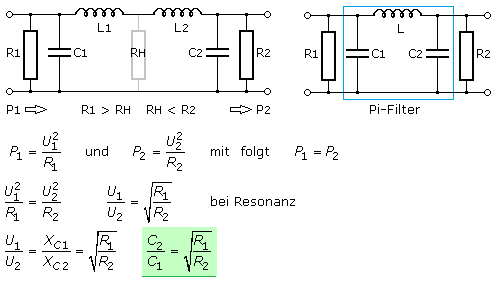In D. Stoll: Introduction to communications engineering, AEG-Telefunken 1979, the following relationships are specified for power adjustment using the Collins filter for dimensioning L and C. A simulation circuit was dimensioned with these equations in order to adapt a low-resistance transmitter antenna to a high-resistance HF tube transmitter for a frequency of 10 MHz and an operating quality of 15. Filters with a high quality of operation have a high selectivity and a small bandwidth, but also greater losses. Usually values ​​between 10 and 20 are chosen.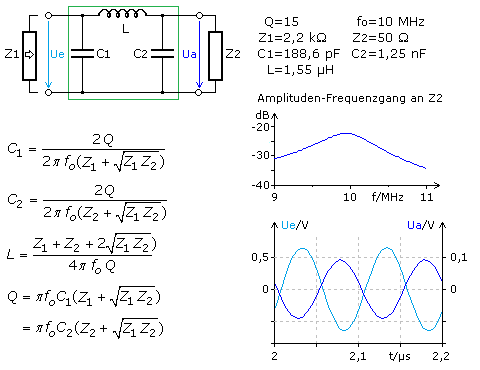In transmitter technology (amateur radio), the capacitors of the Collins filter can be tuned; in some cases, the inductance can also be switched over by taps or tuned as a roller coil. The optimum fine-tuning is then carried out with a standing wave measuring device. The simulation circuit confirms the ratio of C2 / C1 / = 6.63 for the selected load impedances. An optimal power adjustment could not be proven with current and voltage measurements in the simulation. The input voltage Ue at the filter was 30% higher than it should be according to the measured voltage drop at the source internal resistance.

Two filter half-links combined with one another result in a single-link filter chain in a T or PI circuit. A filter optimized for forward operation is operated with power adjustment. The connection resistances at the input and output should correspond to the characteristic impedance of the filter. A well-known application example are audio frequency crossovers. If only the selective property of the filter is important, it is operated with voltage adjustment and loaded as little (high-resistance) as possible. This operating mode can be found in communications and transmission technology with band filters, IF filters and in selective amplifiers. The picture shows simple circuit variants of single-link basic chains and their transmission behavior in the frequency diagram.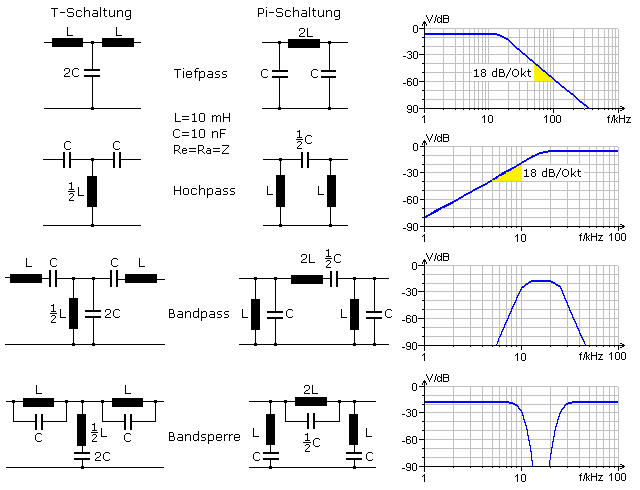The transmission curves show good filter properties. There are no resonance points and the edges of the high and low passes are three times steeper than with simple RC or RL filters at 18 dB per octave. Because of the symmetrical circuit, the input and output impedance depends on the frequency. Power adjustment is almost always chosen for filters in the pass band. An even steeper edge profile is obtained somewhat outside of the power adjustment, whereby different coefficients have proven to be favorable for T and Pi filters.

The following applies to T filters: RL = 0.8 · Z The following applies to pi filters: RL = 1.25 · Z

In the stop band, the input impedance of all T-filters is very high, while it is very small with the pi-filters. Since filter technology is a mathematically demanding and complicated subject, it will not be discussed further here. There is special literature on filter technology; dimensioning can be carried out using filter tables and online calculation programs from the Internet.

### LC band filter for selective amplifiers

In communications technology, certain frequency bands have to be filtered out of a composite signal and processed further. This is done with the help of resonant circuits or filter circuits based on resonant circuits. Since this area is also very extensive and requires a lot of specialist knowledge, only a few important properties are presented here.

### Inductive coupling

In the simplest band filter, two resonant circuits with the same resonance frequency are connected together. The signal coupling takes place inductively according to the transformer principle. The filter characteristics change depending on how close the coils are to one another or the magnetic field of the input circuit is transmitted to the output circuit through a jointly adjustable ferrite core. The transmission curve is determined by the coupling factor and the resonant circuit quality. By tapping the coils according to the autotransformer principle, the signal source in the primary circuit and the load in the secondary circuit can be adjusted so that the circular quality and thus the edge steepness remain high.

The individual circuits, which are shielded from one another, are connected to one another by an additional inductance at the high or top point, referred to ground. Both circuits are also galvanically connected via the coupling inductance. The quotient of the circuit inductance to the coupling inductance determines the strength of the coupling and is inversely proportional to the coupling inductance. It is also dependent on the quality of operation, the ratio of the center frequency to the bandwidth of the filter.

This coupling method is rather unsuitable because, compared to the circular inductance, very large values ​​are required for the coupling inductance and these turn out to be very voluminous. For the simulation band filter examined below, an inductance of 10 mH would be necessary for the critical coupling in the case of inductive head point coupling.

### Inductive base point coupling

The two circles are shielded from each other so that there is no inductive coupling. The coils are tapped with a few turns near their base points and connected to ground with as little loss as possible. The signal current of both circuits then flows through the very small coupling inductance. The critical coupling for the simulation circuit was achieved with 10 nH. The connection resistance had to be less than 2 mΩ for good results.

The two oscillating circuits are shielded from each other. The signals are transmitted via a coupling capacitance in the high point or head point, based on ground. The coupling factor is proportional to the coupling capacitance. For large capacitance values, the capacitor has a low resistance in the frequency band under consideration. The energy transferred to the secondary circuit is higher and the coupling becomes stronger. Small capacitance values ​​have high resistance, less energy is fed to the output circuit and the coupling becomes looser.

### Capacitive base point coupling

The coupling capacitor forms the ground reference of both oscillating circuits. The coupling factor is inversely proportional to the coupling capacitance. In the frequency band, a large capacitance coupling capacitor has a low resistance and transfers less energy to the output circuit. The result is a loose coupling. Smaller capacitor values ​​are higher impedance, the coupling factor is larger and the coupling is stronger.

### Coupling properties

The loose or subcritical coupling is often found with purely inductive coupling. The filter curve is very narrow and pointed and thus resembles the transmission curve of an oscillating circuit of high quality and low bandwidth. The bandwidth is larger for the medium and critical coupling. The filter characteristic also corresponds to that of a resonant circuit with lower damping in the pass band. A fixed or supercritical coupling leads to a broadened transmission curve with a dip in the range of the center frequency. The bandwidth is determined from the height of the saddle point and does not refer to the peak value of the cusp tension.

The two band filters of the simulation circuit are made up of the same individual circuits with a resonance frequency of 503.3 kHz. The internal resistance of the source and the load resistance are equal. If these values ​​are reduced, the bandwidth of the individual circuits increases noticeably and the attenuation increases. The slope of the filter is mainly influenced by the quality of the resonant circuit. In the simulation, the bandwidth is only determined by the degree of coupling. The red curves represent the state of the critical coupling. Comparable coupling factors are calculated for both filter couplings, which in the case of the critical coupling are k ≈ 0.001. Supercritical couplings have larger values ​​and loose couplings have smaller values.### Active filters

The properties of the passive filters are often not sufficient to meet special requirements such as a high edge steepness or selectivity. The signal attenuation within multi-stage passive filters is also a disadvantage. Better results are achieved with active filters. They amplify or weaken defined frequency ranges of the input signal. The inductive assembly (coil) is almost always simulated by an active RC circuit (gyrator). The amplifiers work with frequency-dependent elements in positive or negative feedback circuits.

### Sallen-Key circuit

The active high and low pass filters based on the Sallen-Key principle work with a non-inverting operational amplifier and feedback of the output signal. The frequency-determining elements are in the input and output circuit of the feedback. The gain is Vu = 1 with an impedance converter, but can also be greater with the help of an ohmic voltage divider at the inverting input.

### Multiple feedback circuit

With these active filters with multiple negative feedback, the output signal of the OPV is coupled back to the inverting input on two separate paths. The frequency elements are connected in the input and feedback circuit. The signal amplification is arbitrary.